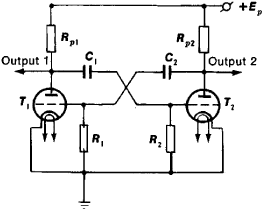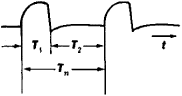# Multivibrator

(redirected from Monoflop)
Also found in: Dictionary.
Related to Monoflop: Bistable circuit

## multivibrator

[‚məl·tə′vī‚brād·ər]
(electronics)
A relaxation oscillator using two tubes, transistors, or other electron devices, with the output of each coupled to the input of the other through resistance-capacitance elements or other elements to obtain in-phase feedback voltage.

## Multivibrator

a relaxation generator of electrical oscillations of the pulsed type; it consists of two amplifiers connected by interstage positive feedback. The term “multivibrator,” whichFigure 1. Circuit diagram of a balanced multivibrator: (Tt) and (T1) tubes (triodes), (RP1 and (RP2 plate circuit loads, (R1) and (R,2) resistances in the feedback circuits, (C1) and (C2) capacitors in the feedback circuits, (EP) plate power supply

was proposed by the Dutch physicist van der Pol, refers to the multiplicity of harmonics in the spectrum of generated oscillations (in this sense a generator of sinusoidal oscillations could be called a monovibrator). The classical multivibrator circuit with two resistance-coupled electron-tube amplifiers with cross-connected plates and grids (see Figure 1) is known as the Abraham-Bloch circuit and is similar to the “cathode relay” circuit proposed by the Soviet scientist M. A. Bonch-Bruevich.

A distinction is made between balanced multivibrators (see Figure 1) and asymmetric multivibrators. In the former case the durations T1 and T2 of the operational cycles, which together make up the oscillation period Tn are equal (see Figure 2); in the latter case they are different. The times T1 and T2 are basically determined by the components R1 and R2, C1 and C2 of the multivibrator.Figure 2. Shape of signal at the output of a multivibrator: (Tn) period of oscillations, (T1) and (T2) durations of operational cycles, (t) time

Many versions of multivibrators exist, using electron tubes, transistors, thyristors, and integrated circuits. Transistor multivibrators are the most widely used. If transistors of the same type are used (p-n-p or n-p-n), the amplifiers of the multivibrator are excited alternately (one amplifier is excited during the time period T1 and the other during the time period T2). Such multivibrators are called bistable multivibrators. The phase alternation is determined by the dynamic condition of the amplifier that is in the unexcited state; it becomes excited when the voltage acting on its input becomes large enough to trigger the shutdown transistor. A short-term regenerative process (during which both amplifiers are excited) takes place subsequently. This process results in a change in the state of the amplifiers—that is, an inversion of the multivibrator. However, if transistors of different types are used in the amplifiers, both amplifiers are excited simultaneously and are in the excited state during the period T1; then they almost simultaneously pass into the unexcited state for the period T2. The transition from the excited state to the unexcited state is determined by the ratio of currents in the collector and base circuits of a saturated amplifier transistor. The principle of operation of such a multivibrator is similar to that of a transistor blocking generator.

Multivibrators are used as pulse generators, frequency dividers, pulse-shaping circuits, and contactless switches for equipment used in automation, computer technology, and measurement technology, such as time relays, drivers, and shapers in digital computers. Like other relaxation oscillators, a multivibrator can operate in both the self-excited mode and delay mode (the latter type is called a flip-flop multivibrator; it is also frequently, but incorrectly, called a monovibrator). Upon receiving a control or starting signal, a flip-flop multivibrator becomes excited and generates one working pulse of duration T1 and then again undergoes a transition to the quiescent state (T2). Flip-flop multivibrators are usually connected in an asymmetric circuit. Their main field of application is the generation of pulses of strictly defined shape.

In addition to two-phase multivibrators, there are also multiphase (n-phase) multivibrators, which consist of n resistance-coupled amplifiers coupled by one common feedback circuit and n interstage feedback circuits. A sequence of pulses that are shifted in time and space can be obtained at the outputs of the n amplifiers of a multiphase multivibrator. For this reason, multiphase multivibrators are frequently used in multichannel systems for selection, transmission, and processing of data.

### REFERENCES

Belen’kii, la. E. Mnogofaznye relaksatory. Kiev, 1966.
Spravochnik po impul’snoi tekhnike, 3rd ed. Edited by V. N. Iakovlev, Kiev, 1972.
Itskhoki, la. S., and N. I. Ovchinnikov. Impul’snye i tsifrovye ustroistva. Moscow, 1972.
Gorokhov, V. A., and M. B. Shchedrin. Fizicheskie osnovy primeneniia tiristorov v impul’snykh skhemakh. Moscow, 1972.
Gorn, L. S., A. A. Klimashov, and B. I. Khazanov. “Mul’tivibratory na integral’nykh elementakh TTL.” Radiotekhnika, 1973, vol. 28, no. 5.

IA. S. ITSKHOKI

## Multivibrator

A form of electronic circuit that employs positive feedback to cross-couple two devices so that two distinct states are possible, for example, one device ON and the other device OFF, and in which the states of the two devices can be interchanged either by use of external pulses or by internal capacitance coupling. When the circuit is switched between states, transition times are normally very short compared to the ON and OFF periods. Hence, the output waveforms are essentially rectangular in form.

Multivibrators may be classified as bistable, monostable, or astable. A bistable multivibrator, often referred to as a flip-flop, has two possible stable states, each with one device ON and the other OFF, and the states of the two devices can be interchanged only by the application of external pulses. A monostable multivibrator, sometimes referred to as a one-shot, also has two possible states, only one of which is stable. If it is forced to the opposite state by an externally applied trigger, it will recover to the stable state in a period of time usually controlled by a resistance-capacitance (RC) coupling circuit. An astable multivibrator has two possible states, neither of which is stable, and switches between the two states, usually controlled by two RC coupling time constants. The astable circuit is one form of relaxation oscillator, which generates recurrent waveforms at a controllable rate.

#### Symmetrical bistable multivibrator

In bistable multivibrators, either of the two devices in a completely symmetrical circuit may remain conducting, with the other nonconducting, until the application of an external pulse. Such a multivibrator is said to have two stable states.

The original form of bistable multivibrator made use of vacuum tubes and was known as the Eccles-Jordan circuit, after its inventors. It was also called a flip-flop or binary circuit because of the two alternating output voltage levels. The junction field-effect transistor (JFET) circuit (Fig. 1) is a solid-state version of the Eccles-Jordan circuit. Its resistance networks between positive and negative supply voltages are such that, with no current flowing to the drain of the first JFET, the voltage at the gate of the second is slightly negative, zero, or limited to, at most, a slightly positive value. The resultant current in the drain circuit of the second JFET causes a voltage drop across the drain load resistor; this drop in turn lowers the voltage at the gate of the first JFET to a sufficiently negative value to continue to reduce the drain current to zero. This condition of the first device OFF and the second ON will be maintained as long as the circuit remains undisturbed. See Transistor

If a sharp negative pulse is applied to the gate of the ON transistor, its drain current decreases and its drain voltage rises. A fraction of this rise is applied to the gate of the OFF transistor, causing some drain current to flow. The resultant drop in drain voltage, transferred to the gate of the ON transistor, causes a further rise at its drain. The action is thus one of positive feedback, with nearly instantaneous transfer of conduction from one device to the other. There is one such reversal each time a pulse is applied to the gate of the ON transistor. Normally pulses are applied to both transistors simultaneously so that whichever device is ON will be turned off by the action. The capacitances between the gate of one transistor and the drain of the other play no role other than to improve the high-frequency response of the voltage divider network by compensating for the input capacitances of the transistors and thereby improving the speed of transition.

A bipolar transistor counterpart of the JFET bistable multivibrator uses npn bipolar transistors. The base of the transistor corresponds to the gate, the emitter to the source, and the collector to the drain. Although waveforms are of the same polarity and the action is roughly similar to that of the JFET circuit, there are important differences. The effective resistance of the base-emitter circuit, when it is forward-biased and being used to control collector current, is much lower than the input gate resistance of the JFET when the latter resistance is used to control drain current (a few thousand ohms compared to a few megohms). This fact must be taken into account when the divider networks are designed. If pnp transistors are used, all voltage polarities and current directions are reversed.

#### Unsymmetrical bistable circuits

Bistable action can be obtained in the emitter- or source-coupled circuit with one of the set of cross-coupling elements removed (Fig. 2). In this case, regenerative feedback necessary for bistable action is obtained by the one remaining common coupling element, leaving one emitter or gate free for triggering action. Biases can be adjusted such that device 1 is ON, forcing device 2 to be OFF. In this case, a pulse can be applied to the free input in such a direction as to reverse the states. Alternatively, device 1 may initially be OFF with device 2 ON. Then an opposite polarity pulse is required to reverse states. Such an unsymmetrical bistable circuit, historically referred to as the Schmitt trigger circuit, finds widespread use in many applications.

#### Monostable multivibrator

A monostable or one-shot multivibrator has only one stable state. If one of the normally active devices is in the conducting state, it remains so until an external pulse is applied to make it nonconducting. The second device is thus made conducting and remains so for a duration dependent upon RC time constants within the circuit itself. Monostable multivibrators are available commercially in integrated chip form. See Integrated circuits

#### Astable multivibrator

The astable multivibrator has capacitance coupling between both of the active devices and therefore has no permanently stable state. Each of the two devices functions in a manner similar to that of the capacitance-coupled half of the monostable multivibrator. It will therefore generate a periodic rectangular waveform at the output with a period equal to the sum of the OFF periods of the two devices.

Astable multivibrators, although normally free-running, can be synchronized with input pulses recurrent at a rate slightly faster than the natural recurrence rate of the device itself. If the synchronizing pulses are of sufficient amplitude, they will bring the internal waveform to the conduction level at an earlier than normal time and will thereby determine the recurrence rate.

#### Logic gate multivibrators

Multivibrators may be formed by using two cross-coupled logic gates, with the unused input terminals used for triggering purposes. The bistable forms of such circuits are usually referred to as flip-flops. See Logic circuits

Site: Follow: Share:
Open / Close